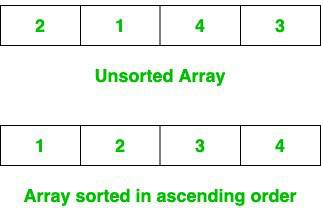Open In App

# C program to sort an array in ascending order

Sorting an array in ascending order means arranging the elements from smallest element to largest element. In this article, we will learn to sort an array in ascending order in the C programming language.There are many ways by which the array can be sorted in ascending order, For simplicity we will be using Selection Sort in this article.

## Algorithm

Selection sort is a simple sorting algorithm that repeatedly finds the minimum element from the unsorted part of the array and places it at the beginning of the sorted part of the array until the complete array is sorted.

1. The array can be sorted in ascending order by repeatedly finding the minimum element (considering ascending order) from the unsorted part and putting it at the beginning.

2. The algorithm maintains two subarrays in a given array.
• The subarray which is already sorted.
• Remaining subarray which is unsorted.

3. In every iteration of the selection sort, the minimum element (considering ascending order) from the unsorted subarray is picked and moved to the sorted subarray.

Refer to the complete article on Selection Sort for more details!

## C

 `// C program to sort the array in an``// ascending order using selection sort``#include `` ` `void` `swap(``int``* xp, ``int``* yp)``{``    ``int` `temp = *xp;``    ``*xp = *yp;``    ``*yp = temp;``}`` ` `// Function to perform Selection Sort``void` `selectionSort(``int` `arr[], ``int` `n)``{``    ``int` `i, j, min_idx;`` ` `    ``// One by one move boundary of``    ``// unsorted subarray``    ``for` `(i = 0; i < n - 1; i++) {``        ``// Find the minimum element in``        ``// unsorted array``        ``min_idx = i;``        ``for` `(j = i + 1; j < n; j++)``            ``if` `(arr[j] < arr[min_idx])``                ``min_idx = j;`` ` `        ``// Swap the found minimum element``        ``// with the first element``        ``swap(&arr[min_idx], &arr[i]);``    ``}``}`` ` `// Function to print an array``void` `printArray(``int` `arr[], ``int` `size)``{``    ``int` `i;``    ``for` `(i = 0; i < size; i++)``        ``printf``(``"%d "``, arr[i]);``    ``printf``(``"\n"``);``}`` ` `// Driver code``int` `main()``{``    ``int` `arr[] = { 0, 23, 14, 12, 9 };``    ``int` `n = ``sizeof``(arr) / ``sizeof``(arr);``    ``printf``(``"Original array: \n"``);``    ``printArray(arr, n);`` ` `    ``selectionSort(arr, n);``    ``printf``(``"\nSorted array in Ascending order: \n"``);``    ``printArray(arr, n);`` ` `    ``return` `0;``}`

Output

```Original array:
0 23 14 12 9

Sorted array in Ascending order:
0 9 12 14 23 ```

### Complexity Analysis

• Time Complexity: O(N2)
• Auxiliary Space: O(1)